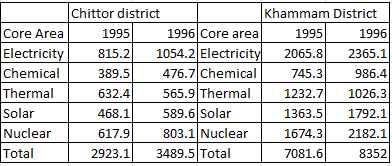### CAT 1996 Question Paper Question 62

Instructions

Answer the questions based on the following information. The amount of money invested (rupees in crores) in the core infrastructure areas of two districts, Chittoor and Khammam, Andhra Pradesh, is as follows.Question 62

# The investment in electricity and thermal energy in 1995 in these two districts formed what per cent of the total investment made in that year?

Solution

Total investment in year $$1995 = 7081.6 + 2923.1 = 10004.7$$
Total electricity and thermal invesment in year 1995 for both villages = $$815.2 + 632.4 + 2065.8 + 1232.7 = 4726.1$$
Percentage investment = $$\frac{4726.1}{10004.7} \times 100$$
Through approximation method, we can say that above value will be less than 50 and more than 47
Hence among all options, B will be most appropriate answer.

• All Quant CAT Formulas and shortcuts PDF
• 30+ CAT previous papers with solutions PDF